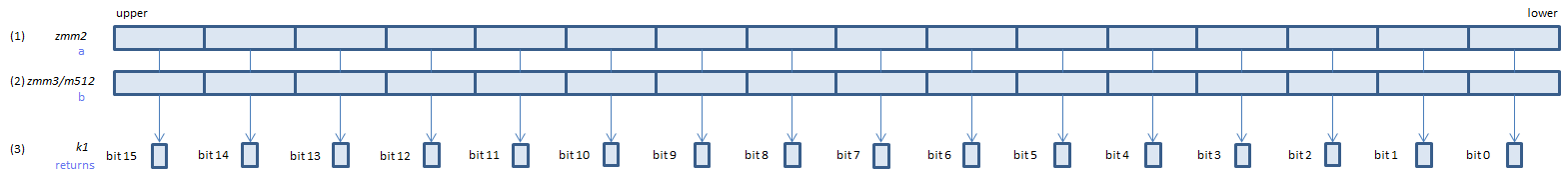﻿ cmpneqps

## CMPNEQPS - CoMPare Not EQual Packed Single

CMPNEQPS xmm1, xmm2/m128    (S1
__m128 _mm_cmpneq_ps(__m128 a, __m128 b)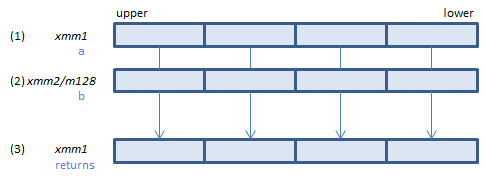For each float, if (1) != (2) or either or both of (1)(2) is NaN, set 1, else set 0, to all bits of the corresponding float of (3).
VCMPNEQPS xmm1, xmm2, xmm3/m128    (V1
__m128 _mm_cmpneq_ps(__m128 a, __m128 b)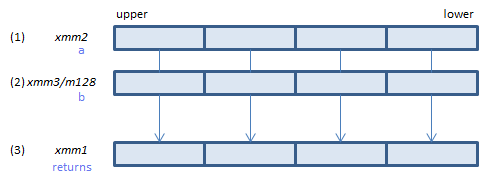For each float, if (1) != (2) or either or both of (1)(2) is NaN, set 1, else set 0, to all bits of the corresponding float of (3).
VCMPNEQPS ymm1, ymm2, ymm3/m256    (V1
__m256 _mm256_cmp_ps(__m256 a, __m256 b, _CMP_NEQ_UQ)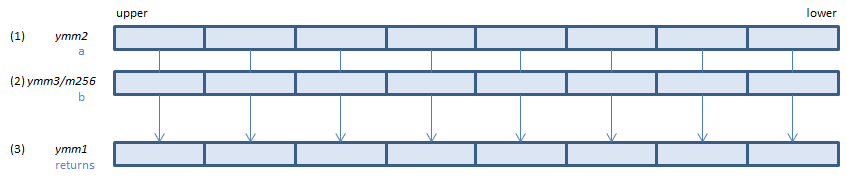For each float, if (1) != (2) or either or both of (1)(2) is NaN, set 1, else set 0, to all bits of the corresponding float of (3).
VCMPNEQPS k1{k2}, xmm2, xmm3/m128/m32bcst    (V5+VL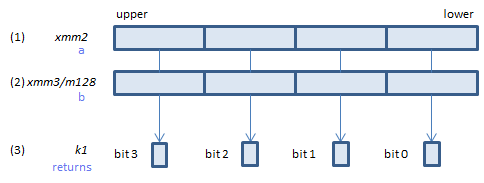For each float, if (1) != (2) or either or both of (1)(2) is NaN, set 1, else set 0, to the corresponding bit of (3).
If k2 bit is 0, the comparison is not done and the corresponding bit of (3) is set to zero. Upper bits of (3) are zero cleared.
VCMPNEQPS k1{k2}, ymm2, ymm3/m256/m32bcst    (V5+VL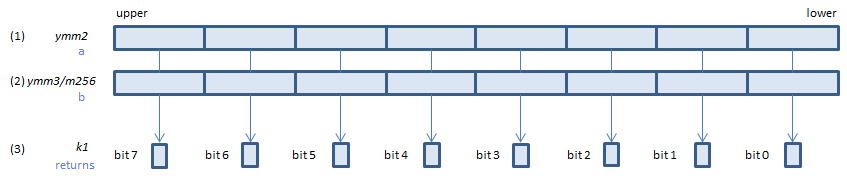For each float, if (1) != (2) or either or both of (1)(2) is NaN, set 1, else set 0, to the corresponding bit of (3).
If k2 bit is 0, the comparison is not done and the corresponding bit of (3) is set to zero. Upper bits of (3) are zero cleared.
VCMPNEQPS k1{k2}, zmm2, zmm3/m512/m32bcst{sae}    (V5